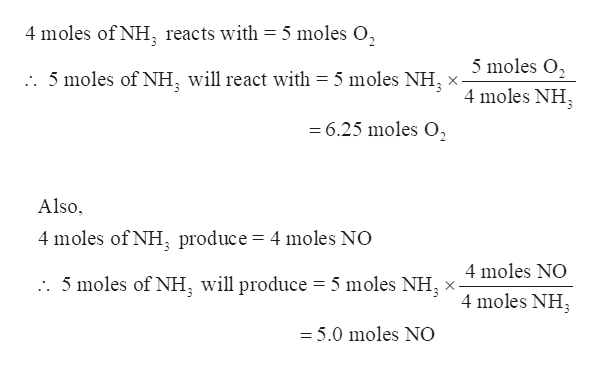# The first step in the Ostwald process for making nitric acid is the formation of NO according to: 4(NH3) + 5(O2) --> 4(NO) + 6(H2O)5 moles of NH3 will react stoichiometrically with _____ moles of O2 to form ______ moles of NO.

Question
39 views

The first step in the Ostwald process for making nitric acid is the formation of NO according to: 4(NH3) + 5(O2) --> 4(NO) + 6(H2O)

5 moles of NH3 will react stoichiometrically with _____ moles of O2 to form ______ moles of NO.

check_circle

Step 1

The first step in the Ostwald process for making nitric acid is the formation of NO according to:

4(NH3) + 5(O2) →4(NO) + 6(H2O)

The moles of O2 required by 5 moles of NH3 and also the moles of NO produced are to be calculated.

Step 2

As per the balanced equation, 4 moles of NH3 reacts with 5 moles of O2 to fo...help_outlineImage Transcriptionclose4 moles of NH, reacts with = 5 moles O, 5 moles O2 2. 5 moles of NH, will react with 5 moles NH 4 moles NH 6.25 moles O Also, 4 moles of NH produce = 4 moles NO 4 moles NO . 5 moles of NH, will produce = 5 moles NH 4 moles NH =5.0 moles NO fullscreen

### Want to see the full answer?

See Solution

#### Want to see this answer and more?

Solutions are written by subject experts who are available 24/7. Questions are typically answered within 1 hour.*

See Solution
*Response times may vary by subject and question.
Tagged in
ScienceChemistry

### General Chemistry